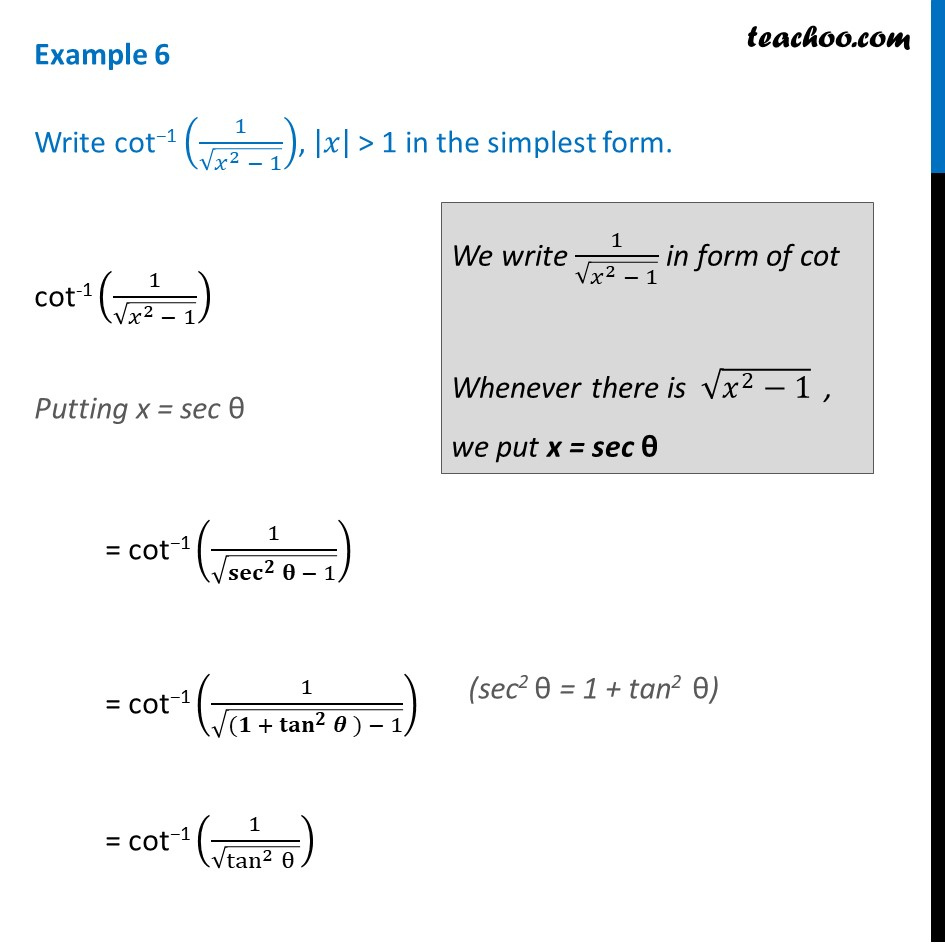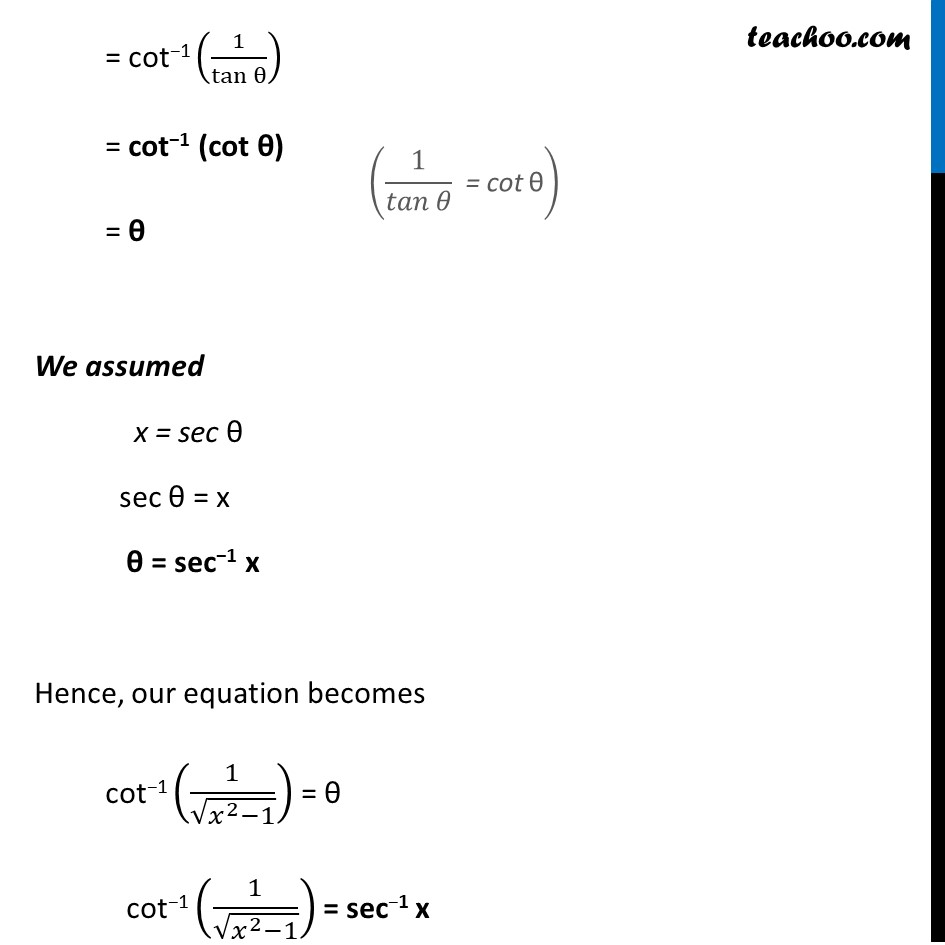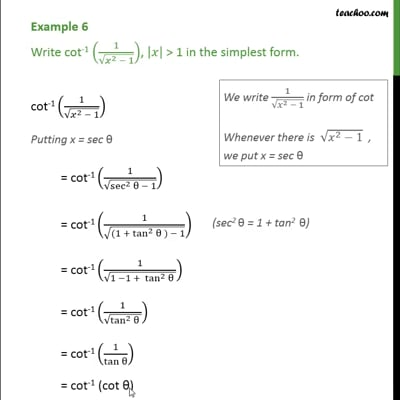Not clear how to approach

Chapter 2 Class 12 Inverse Trigonometric Functions
Concept wiseThis video is only available for Teachoo black users

Introducing your new favourite teacher - Teachoo Black, at only ₹83 per month

### Transcript

Example 6 Write cot−1 (1/√(𝑥^2 − 1)), |𝑥| > 1 in the simplest form. cot-1 (1/√(𝑥^2 − 1)) Putting x = sec θ = cot−1 (1/√(〖𝐬𝐞𝐜〗^𝟐⁡𝛉 − 1)) = cot−1 (1/√(〖(𝟏 + 〖𝐭𝐚𝐧〗^𝟐〗⁡𝜽 ) − 1)) = cot−1 (1/√(tan^2⁡θ )) We write 1/√(𝑥^2 − 1) in form of cot Whenever there is √(𝑥^2−1) , we put x = sec θ = cot−1 (1/tan⁡θ ) = cot−1 (cot θ) = θ We assumed x = sec θ sec θ = x θ = sec−1 x Hence, our equation becomes cot−1 (1/√(𝑥^2−1)) = θ cot−1 (1/√(𝑥^2−1)) = sec−1 x